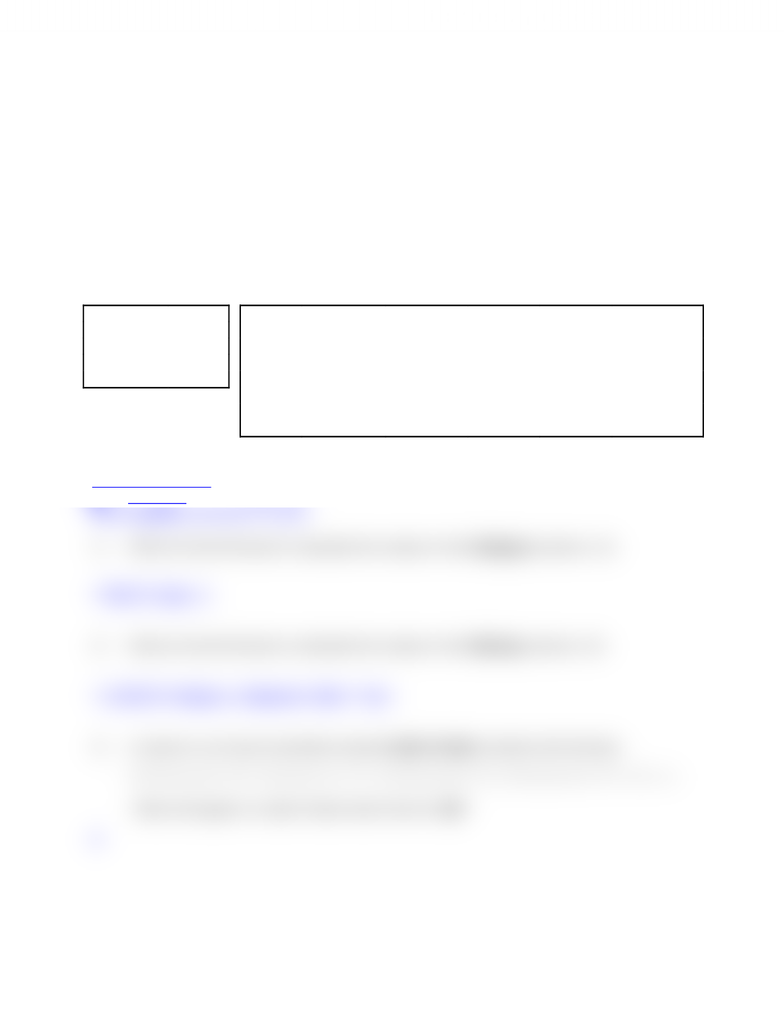# Test 1 version 2 part b

26 views1 pages
School
York University
Department
Electrical Engineering and Computer Science
Course
EECS 1520Part B – Construct Excel formulas [8 points]
Use only the data shown to answer the following questions.
All ranges have been named using the labels that appear in the worksheets.
The graphics below are taken from an Inventory/Sales system.
The left worksheet includes parameters:
Categories are used to select the Rate to apply to calculate Markup.
In the right worksheet:
Code and Cost hold data entered by the user.
Code includes the category of each item as the last
character
and the Stockroom number as the last
digit
.
Category, Markup, Price, and Stockroom are calculated.
Categories Rate
B 100%
G 125%
M 110%
W 150%
Code Cost Category Markup Price Stockroom
123W \$ 3.40 W \$ 5.10 \$ 8.50 3
254B \$ 9.84 B \$ 9.84 \$ 19.68 4
256W \$ 5.60 W \$ 8.40 \$ 14.00 6
255G \$ 12.39 G \$ 15.49 \$ 27.88 5
274B \$ 4.49 B \$ 4.49 \$ 8.98 4
358M \$ 0.95 M \$ 1.05 \$ 2.00 8
568M \$ 9.52 M \$ 10.47 \$ 19.99 8
Notes to Markers:
Each underline represents 1 point.
Each underline represents ½ point.
1) Write an Excel formula to calculate the values in the Category column. 
= RIGHT (Code, 1)
2) Write an Excel formula to calculate the values in the Markup column. 
= LOOKUP (Category, Categories, Rate) * Cost
3) A column in an Excel worksheet named Letter Grade contains this formula:
=IF(Score<80,"B",IF(Score<70,"C",IF(Score<60,"D",IF(Score<50,"F","A"))))
What will appear in Letter Grade when Score is 56?
B
Unlock document

This preview shows half of the first page of the document.
Unlock all 1 pages and 3 million more documents.

## Document Summary

Part b construct excel formulas [8 points] Use only the data shown to answer the following questions. All ranges have been named using the labels that appear in the worksheets. The graphics below are taken from an inventory/sales system. Categories are used to select the rate to apply to calculate markup. Code and cost hold data entered by the user. Code includes the category of each item as the last character and the stockroom number as the last digit. Category, markup, price, and stockroom are calculated. Each underline represents point: write an excel formula to calculate the values in the category column. = right (code, 1: write an excel formula to calculate the values in the markup column. A column in an excel worksheet named letter grade contains this formula: